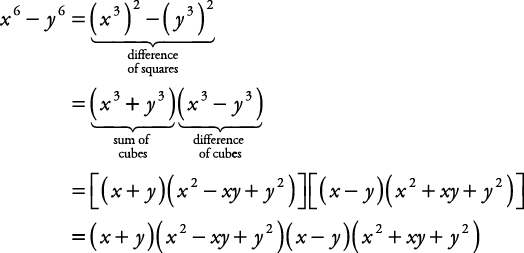## Sum or Difference of Cubes

A polynomial in the form a 3 + b 3 is called a sum of cubes. A polynomial in the form a 3b 3 is called a difference of cubes.

Both of these polynomials have similar factored patterns:

• A sum of cubes: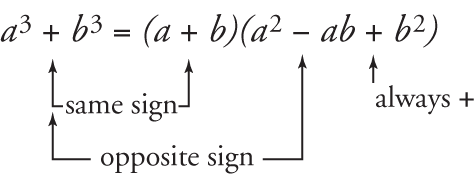• A difference of cubes:##### Example 1

Factor x 3 + 125.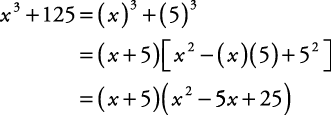##### Example 2

Factor 8 x 3 – 27.##### Example 3

Factor 2 x 3 + 128 y 3.

First find the GCF. GCF = 2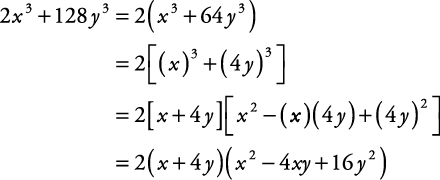##### Example 4

Factor x 6y 6.

First, notice that x 6y 6 is both a difference of squares and a difference of cubes.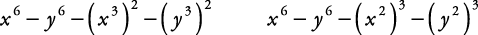In general, factor a difference of squares before factoring a difference of cubes.